# Collision between two uniformly moving particles

brotherbobby
Homework Statement:
Shown in the picture below, two particles ##1## and ##2## are set into motion simultaneously from the edge of a table with velocities ##v_1## and ##v_2## at angles ##\theta_1## and ##\theta_2##. If their initial distance of separation is ##\boldsymbol{s}##, answer the following questions :
1. What is the necessary condition for ##1## and ##2## to collide?
2. Prove that for collision to be, the relative velocity is also the velocity of approach.
3. Find the time after which collision occurs.
Relevant Equations:
1. For two particles of the given type to ##\textbf{collide}##, there should be ##\textbf{no motion}## perpendicular to the line joining them. This implies that one particle would "see" the other move along the line joining them.
2. Relative velocity between the two particles ##v_{R} = (v_1^2+v_2^2+2v_1v_2\cos({\theta_1+\theta_2}))^{1/2}##. (You may need to draw the diagram to convince yourself of this - it's not trivial).
3. For the two given particles, the velocity of approach ##v_A = v_1\cos\theta_1+v_2\cos\theta_2 = -\frac{ds}{dt}##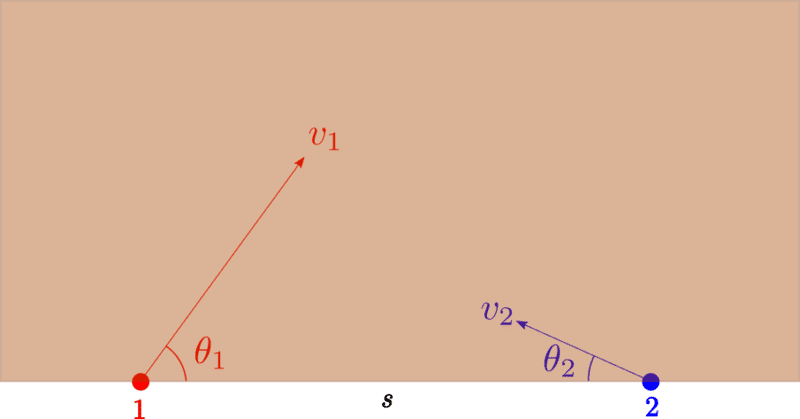Problem :
The statement is given above. Here I attach the image of the problem to the right.

Attempt :

(1) Condition for collision : For the two particles to collide, there should be no relative velocity perpendicular to their "separation vector" ##\boldsymbol{s}##. Hence, we must have : ##\boxed{v_1 \sin\theta_1=v_2\sin\theta_2}## ##\huge{\checkmark}## (agrees with book)

(2) For collision, ##\boldsymbol{v_R = v_A}## : While I understand that the author's demand is a mathematical one, it can be show intuitively too. If the two particles possesses no relative velocity perpendicular to their separation vector ##\boldsymbol{s}##, the only other component of relative velocity is along their separation vector. But that is also the velocity of approach - proving the supposition. ##\\[10pt]##
Now for the same result mathematically. We have the following three relations :
\begin{equation}
v_1\sin\theta_1 = v_2\sin\theta_2
\end{equation}
\begin{equation}
v_R^2 = v_1^2+v_2^2+2v_1v_2\cos(\theta_1+\theta_2)
\end{equation}
\begin{equation}
v_A = v_1\cos\theta_1+v_2\cos\theta_2
\end{equation}
Squaring the last equation, we get
\begin{equation}
v_A^2=v_1^2\cos^2\theta_1+v_2^2\cos^2\theta_2+2v_1v_2\cos\theta_1\cos\theta_2
\end{equation}
Squaring (1), we get $$v_1^2\sin^2\theta_1=v_2^2\sin^2\theta_2\Rightarrow v_1^2-v_1^2\cos^2\theta_1 = v_2^2-v_2^2\cos^2\theta_2\Rightarrow v_1^2\cos^2\theta_1 = v_1^2-v_2^2+v_2^2\cos^2\theta_2$$
Substituting the value of ##v_1^2\cos^2\theta_1## into (4), we get
$${v_A^2 = v_1^2-v_2^2+2v_2^2\cos^2\theta_2+2v_1v_2\cos\theta_1\cos\theta_2\Rightarrow v_A^2=\underbrace{v_1^2+v_2^2+2v_1v_2\cos(\theta_1+\theta_2)}_{v_R^2}-2v_2^2+2v_2^2\cos^2\theta_2+2v_1v_2\sin\theta_1\sin\theta_2,}$$
where I have "forced" ##v_R^2## to appear on the right and had to subtract and add terms accordingly to make up for it.
Taking ##-2v_2^2## out as common and remembering that ##v_1 \sin\theta_1=v_2\sin\theta_2##, the last equation simplifies to
$$v_A^2 = v_R^2 -\cancel{2v_2^2\sin^2\theta_2}+\cancel{2v_2\sin^2\theta_2}= v_R^2\Rightarrow v_A = \pm v_R.$$
The relative velocity and the velocity of approach ##v_R, v_A\ge 0##. If two particles are moving "away" from one another, we would have ##v_A = +\dfrac{ds}{dt}##. Hence, our answer is ##\boxed{v_R=v_A}##; additionally, ##\boxed{v_A = -\dfrac{ds}{dt}}##, as particles 1 and 2 move "towards" one another, implying that their distance of separation decreases with time.

(3) Time of collision : The timeat which collision would occur is : ##\boxed{t = \dfrac{s}{v_1\cos\theta_1+v_2\cos\theta_2}}## ##\huge{\checkmark}## (agrees with book)

Though I have been correct I think, I would appreciate if point no. 2 above, where ##v_A = v_R## could have been done better. Or any other comment when it comes to the "velocity of approach" as against the "relative velocity" between two vectors.

Last edited:
•Delta2

Homework Helper
Gold Member
You can do it formally with vectors. You know that
##\vec v_1 = v_1\cos\theta_1~\hat x+ v_1\sin\theta_1~\hat y## and that ##\vec v_2 = -v_2\cos\theta_2~\hat x+ v_2\sin\theta_2~\hat y##.
1. Use these to find an expression for the relative velocity ##\vec v_{\text{12}}=\vec v_1-\vec v_2## and set it aside.
2. Write expressions for the displacement of each particle relative to its starting point in time ##\Delta t##, ##\Delta\vec s_1=\vec v_1\Delta t## and ##\Delta\vec s_2=\vec v_2\Delta t##.
3. Find the relative displacement, ##\Delta\vec s_{\text{12}}=\Delta\vec s_1-\Delta\vec s_2.##
4. The velocity of approach is a measure of how fast the distance between the two is shrinking, ##\vec v_{\text{app.}}=\dfrac{\Delta\vec s_{\text{12}}}{\Delta t}.##
5. Compare it with the expression for the relative velocity ##\vec v_{\text{12}}## that you found earlier.

brotherbobby
Thank you @kuruman . Let me try it out.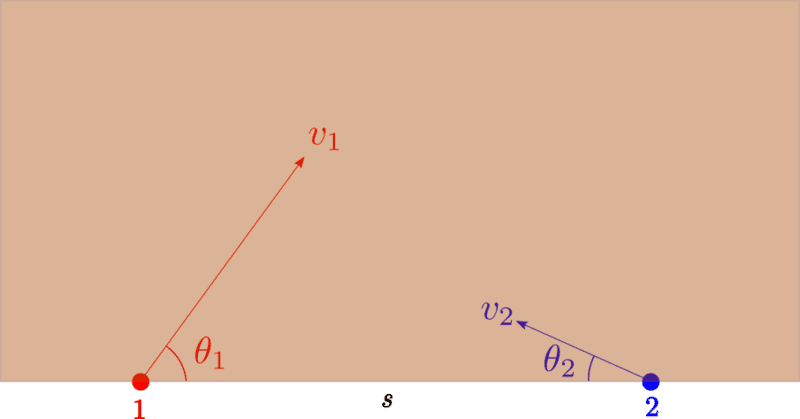Question :
I start by putting the image of the problem. The main question is to prove that in the event of a collision, the velocity of approach is the relative velocity ##\boxed{\boldsymbol{\vec v_A=\vec v_R}}##.

Attempt : I agree, as you have said, that the velocities of the particles are the following : ##\vec v_1 = v_1\cos\theta_1\hat x+v_1\sin\theta_1\hat y## and ##\vec v_2 = -v_2\cos\theta_2\hat x+v_2\sin\theta_2\hat y##.

(1) The relative velocity of particle 1 relative to particle 2 : ##\vec{v}_{12} = \vec v_1-\vec v_2 = (v_1\cos\theta_1+ v_2\cos\theta_2)\hat x+(v_1\sin\theta_1-v_2\sin\theta_2)\hat y##.

At this stage, upon working out what you had asked of me in your points 2,3,4 and 5 above, I find that it amounts to ##\vec v_{\text{app}} = \vec v_{12}##. In this problem that is true, but it is not generally. The velocity of approach varies between two particles from point to point as the particles cover ground, unlike relative velocity. Only when particles collide, which is what happens in this case, does the velocity of approach remain the same, equal to the relative velocity.

So what is the velocity of approach, generally? It is the velocity with which one particle approaches another, along the line that joins them, at a given instant. Generally, ##v_{\text{app}} = v_1\cos\theta_1+v_2\cos\theta_2 \ne \vec{v}_{12}##.

I do not want to waste time typing it out here in MATH mode, but let me copy and paste work below which I did using AUTODESK SKETCHBOOK. I begin from point(2) onwards, referring to your post#2 above.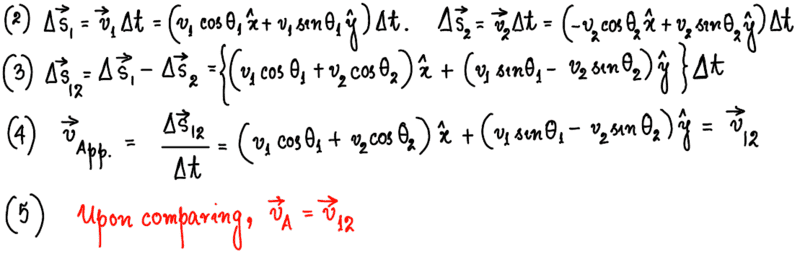Clearly, the strategy for finding the velocity of approach ##\vec v_{\text{app}}## is mistaken. I need to think in order to find a vector method of finding it.

Homework Helper
Gold Member
First let me say that your Autodesk sketchbook output is just fine and clear to read.

Secondly, question 2 asks, "Prove that for collision to be, the relative velocity is also the velocity of approach." You already know that, for the collision to be, the necessary condition is ##v_1\sin\theta_1 = v_2\sin\theta_2.## If you put that in your expressions, you get ##\vec v_{\text{app.}}=(v_1\cos\theta_1 + v_2\cos\theta_2)~\hat x.## That is a vector allong the line joining the masses at all times which in this case is the x-axis.

Thirdly, I am not sure what you mean by needing to find a vector method. Why isn't what you have in post #3 vector method? Did you mean a drawing with arrows instead of an equation with unit vectors? Perhaps you are misled by the drawing you posted because it is highly inaccurate. A diagram drawn to scale often clarifies and focuses one's thoughts (see below.)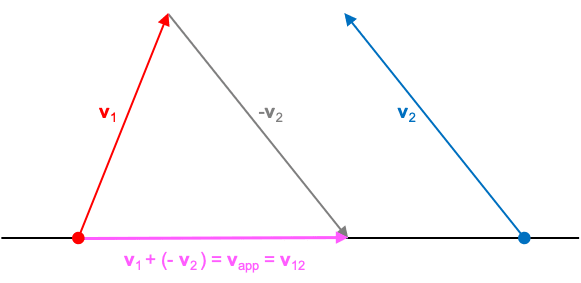Last edited:
brotherbobby
If you put that in your expressions, you get v→app.=(v1cos⁡θ1+v2cos⁡θ2) x^.
That is correct, however the expression I have written out in (4) in my post # 3 above is not the velocity of approach. Let me type that out for you for easy reference. $$\vec v_{\text{app}}= (v_1\cos\theta_1+v_2\cos\theta_2)\hat x+(v_1\sin\theta_1-v_2\sin\theta_2)\hat y.$$ It is the expression for relative velocity which is a constant ##\vec v_{12}=\vec v_1-\vec v_2##. On the other hand, the velocity of approach should be a function of time! It changes at every instant when particles 1 and 2 have moved a (small) distance. Let me draw in AUTODESK SKETCHBOOK to show you what I mean. Of course the vector expression of ##\vec v_{\text{app}}## is still something I have to think about. I will try it after the drawing which I paste below.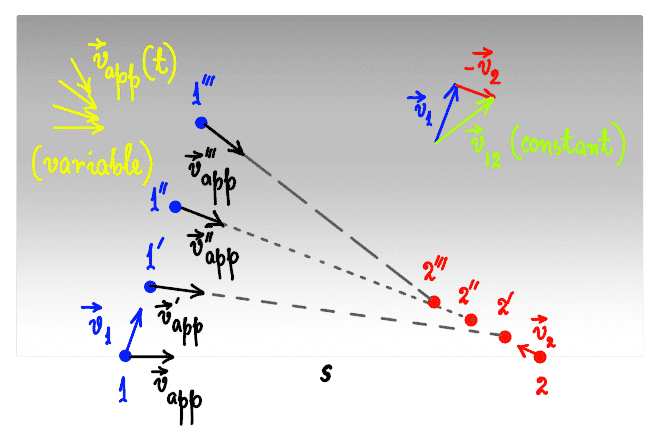I have drawn this diagram for the general case, not for this problem condition where the two particles collide. The successive positions of particles 1 and 2 are (say) at every 1 s. Since each particle has a different velocity, they cover different grounds at every equal interval. It is evident from the diagram that ##\vec v_{\text{app}}(t)## is a function of time. In fact, for this pair of particles, we will have the speed of the approach velocity ##|\vec v_{\text{app}}|## constantly decreasing in time, as you will realize. It's angle to the horizontal is 0 at first and increasingly negative. But what is its vectorial form, mathematically?

Let the separation vector from ##2\rightarrow 1## be ##\vec s##. We assume the position of particle 2 to be the origin O. After a time ##t##, we have the particle 1 at ##\vec s_{1}(t)=\vec s + \vec v_1 t## and the position of particle 1 at ##\vec s_{2}(t)=\vec v_2 t##. The displacement vector of 1 relative to 2 at a time ##t## is given by ##s_{12}(t) = \vec s+\vec v_1 t-\vec v_2t##. The approach velocity is directed along this line, from particle ##2\rightarrow 1##. It is harder to proceed from here, using vector addition (or subtraction). Each time, the two vectors ##v_1## and ##v_2## will have to resolved along the displacement vector at that instant.

Homework Helper
Gold Member
I didn't realize that you changed the premise of the problem and that the collision condition was removed. You have ##\vec s_{12}(t) = \vec s+(\vec v_1 -\vec v_2)t=\vec s+\vec v_{12} t## and you want the component of ##\vec v_{12}## in the direction of ##\vec s_{12}.## For that, you need a unit vector in the direction of ##\vec s_{12}##. An arbitrary unit vector is the vector divided by its magnitude. In this case, $$\hat s_{12}=\frac{\vec s_{12}}{|\vec s_{12}|}=\frac{\vec s+(\vec v_1 -\vec v_2)t}{\sqrt{[\vec s+(\vec v_1 -\vec v_2)t]\cdot[\vec s+(\vec v_1 -\vec v_2)t]}}.$$ The approach velocity would be the component of ##\vec v_{12}## in the direction of ##\vec s_{12}## : $$\vec v_{\text{app.}}=\vec v_{12}\cdot\hat s_{12}=\frac{(\vec v_1 -\vec v_2) \cdot [\vec s+(\vec v_1 -\vec v_2)t]}{\sqrt{[\vec s+(\vec v_1 -\vec v_2)t]\cdot[\vec s+(\vec v_1 -\vec v_2)t]}}.$$

brotherbobby
I didn't realize that you changed the premise of the problem and that the collision condition was removed. You have ##\vec s_{12}(t) = \vec s+(\vec v_1 -\vec v_2)t=\vec s+\vec v_{12} t## and you want the component of ##\vec v_{12}## in the direction of ##\vec s_{12}.## For that, you need a unit vector in the direction of ##\vec s_{12}##. An arbitrary unit vector is the vector divided by its magnitude. In this case, $$\hat s_{12}=\frac{\vec s_{12}}{|\vec s_{12}|}=\frac{\vec s+(\vec v_1 -\vec v_2)t}{\sqrt{[\vec s+(\vec v_1 -\vec v_2)t]\cdot[\vec s+(\vec v_1 -\vec v_2)t]}}.$$ The approach velocity would be the component of ##\vec v_{12}## in the direction of ##\vec s_{12}## : $$\vec v_{\text{app.}}=\vec v_{12}\cdot\hat s_{12}=\frac{(\vec v_1 -\vec v_2) \cdot [\vec s+(\vec v_1 -\vec v_2)t]}{\sqrt{[\vec s+(\vec v_1 -\vec v_2)t]\cdot[\vec s+(\vec v_1 -\vec v_2)t]}}.$$
Thank you @kuruman - this is exactly what I was looking for. During our school years we were not taught of the concept of a velocity of approach. Apparently, problems you can do using relative velocity can be done using the approach velocity also. It's all a learning. Thank you for your time.

•kuruman